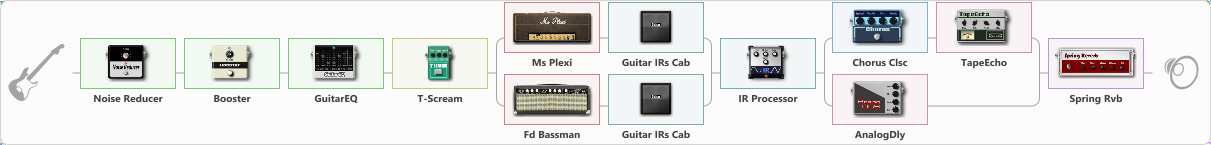# Plexi boost sound

Discussion in 'ToneLib-GFX presets' started by truss van halen, Nov 24, 2020.

1. ### truss van halenWell-Known Member

Plexi boost sound

Preset name: Plexi bust sound

Effects chain:Effect: "Noise Reducer" (Dynamics / Filter), active - "yes"
{
"Sens" = 100
"Mode" = Soft
}

Effect: "Booster" (Dynamics / Filter), active - "yes"
{
"Gain" = 64
}

Effect: "GuitarEQ" (Dynamics / Filter), active - "yes"
{
"160 Hz" = -6
"400 Hz" = 8
"800 Hz" = 9
"1.6 kHz" = -5
"3.2 kHz" = 2
"6.4 kHz" = 1
"12 kHz" = 0
"Level (dB)" = 4
}

Effect: "T-Scream" (Overdrive / Distortion), active - "yes"
{
"Drive" = 79
"Tone" = 80
"Level" = 79
}

Effect: "Splitter" (Dynamics / Filter), active - "yes"
{
"A-Bypass" = Off
"A-Pan" = 0
"A-Level" = 55
"B-Bypass" = Off
"B-Pan" = 0
"B-Level" = 55

'A' branch:
{

Effect: "Ms Plexi" (Amp simulators), active - "yes"
{
"Gain" = 78
"Bass" = 50
"Middle" = 50
"Treble" = 50
"Presence" = 50
"Master" = 61
"Level (dB)" = 2
}

Effect: "Guitar IRs Cab" (Cabinets), active - "yes"
{
"Model" = Fender Deluxe Rev (1x12")
"Mic Position" = Middle
"Mic Distance" = Near
"Low Cut (Hz)" = 60
"Hi Cut (kHz)" = 20.0
"Mix" = 100
"Level (dB)" = 0
}
}
'B' branch:
{

Effect: "Fd Bassman" (Amp simulators), active - "yes"
{
"Gain" = 72
"Bass" = 50
"Middle" = 73
"Treble" = 75
"Presence" = 50
"Master" = 56
"Level (dB)" = 4
}

Effect: "Guitar IRs Cab" (Cabinets), active - "yes"
{
"Model" = Fender Deluxe Rev (1x12")
"Mic Position" = Middle
"Mic Distance" = Near
"Low Cut (Hz)" = 60
"Hi Cut (kHz)" = 12.5
"Mix" = 73
"Level (dB)" = 0
}
}
}

Effect: "IR Processor" (Cabinets), active - "yes"
{
"IR" = Fender Tweed Champ 58
"Low Cut (Hz)" = 0
"Hi Cut (kHz)" = 20.0
"Mix" = 100
"Level (dB)" = 2
}

Effect: "Splitter" (Dynamics / Filter), active - "yes"
{
"A-Bypass" = Off
"A-Pan" = 0
"A-Level" = 55
"B-Bypass" = Off
"B-Pan" = 0
"B-Level" = 55

'A' branch:
{

Effect: "Chorus Clsc" (Modulation / Sfx), active - "yes"
{
"Speed" = 2.9
"Depth" = 24
"Center" = 1.0
"Mode" = Stereo
}

Effect: "TapeEcho" (Delay), active - "yes"
{
"Time" = 316
"Feedback" = 38
"Tone" = 65
"LoDamp" = 19
"Mix" = 80
}
}
'B' branch:
{

Effect: "AnalogDly" (Delay), active - "yes"
{
"Time" = 428
"Feedback" = 60
"Tone" = 68
"Mix" = 66
}
}
}

Effect: "Spring Rvb" (Reverberation), active - "yes"
{
"Time" = 4.3
"PreDelay" = 0
"LoDamp" = 16
"HiDamp" = 26
"Mix" = 36
}

Note: You will need to download and install the ToneLib-GFX software to use the preset.

#### Attached Files:

• ###### Plexi_boost_sound.tlgfx
File size:
1.9 KB
Views:
2,377
Skeve Nelis, Jack Disney and arsser like this.